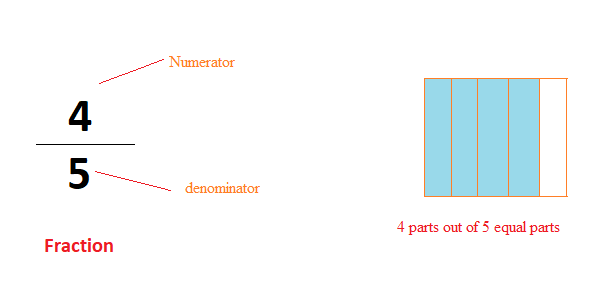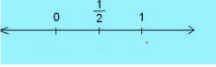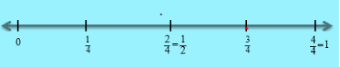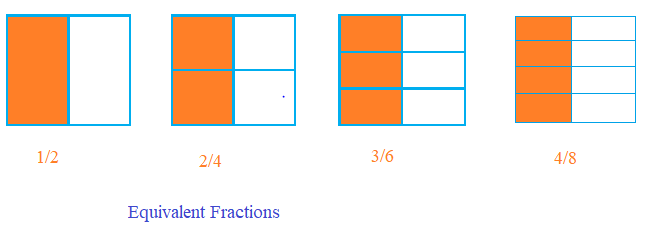# CBSE Notes for Class 6 Maths Chapter 7: Fractions

Table of Content

## Fraction Definition

A fraction is a number representing a part of a whole. The whole may be a single object or a group of objects.
Suppose Ramesh has a chocolate and we want to equally share with his Friend Amit. He will divide the chocolate into two pieces and keep one piece with him and give another piece to Amit. So basically they each have got 1 part out of 2 parts i.e 1/2 of the chocolate. Similarly if they have another suresh also, then they will divide chocolate in three equal parts,then each of them will have 1 out of 3 parts i.e 1/3 of the chocolate
Example
$\frac {3}{11}$
3 out of 11 parts
3 -> Called Numerator
11 -> Called DenominatorImportant Note:
When expressing a situation of counting parts to write a fraction, it must be ensured that all parts are equal
Identify the Numerator and Denominator from below fraction
a. $\frac {2}{15}$
b. $\frac {11}{13}$
c. $\frac {3}{2}$
d. $\frac {1}{8}$

## Types of Fraction

Fractions are of three types.
a. Proper Fraction
b. Improper Fraction
c. Mixed Fraction.
Let dive into each of them in detail in below

## What is proper Fraction

Proper Fraction is the fraction which is less than 1 or where Numerator is less than Denominator
Here the Denominator shows the part whole has been divided and numerator shows the part which has been considered.
This is the same fraction which we discussed with fraction definition
Example
$\frac {1}{3}$
$\frac {2}{3}$
$\frac {4}{5}$

## What is Improper Fraction

Improper Fraction is the fraction which is greater than 1 or where Numerator is greater than Denominator
Lets understand this with example . Suresh has 5 chocolates and he has to divide those chocolate among four friends.We can divide each chocolate into fours parts and each one can have one-quarter part of the each chocolate. So Each friend will be having 5 parts of the one-quarter part.Now 4 parts make one whole. So basically each one of them is getting 1 whole and 1 part. So this can be written as 5/4
Here numerator is more than denominator
Example
$\frac {11}{5}$
$\frac {5}{4}$
$\frac {10}{9}$

## What is Mixed Fraction

It is combination of whole number and proper fraction
$8 \frac {4}{9}$

Lets the example of improper fraction only. The division can made in another way. We give one chocolate to each of them and divide the fifth chocolate into four pieces. So each of them got 1 full chocolate and one-quarter part of last chocolate. So this can written as
$1 + \frac {1}{4} =1 \frac {1}{4}$
This is mixed fraction.
Also $1 + \frac {1}{4} =1 \frac {1}{4} = \frac {5}{4}$
Example
$2 \frac {1}{5}$
$1 \frac {3}{4}$
$1 \frac {1}{9}$

## How to convert Mixed Fractions to Improper Fractions

 Step 1 Obtain the mixed fraction. Let the mixed fraction be 52/6 Step 2 Identify the whole number and the numerator (top) and denominator (bottom) of the proper fraction. Whole Number=5 Numerator=2 Denominator=6 Step 3 Apply the formula $\frac {(Whole \times Denominator) + Numerator}{Denominator}$ Example $5\frac {2}{6}$ =$\frac {32}{6}$
Example
$1\frac {1}{3}$
Apply the formula
$\frac {(Whole \times Denominator) + Numerator}{Denominator}$
So $1\frac {1}{3}=\frac {4}{3}$

Practice Questions
• $1 \frac {1}{4}$
• 5$\frac {2}{3}$
• 3$\frac {5}{11}$
• 2$\frac {17}{44}$
• 1$\frac {3}{7}$
• 3$\frac {5}{9}$

## How to convert Improper Fractions to Mixed Fraction

 Step 1 Obtain the improper fraction. Step 2 Divide the numerator by the denominator and obtain the quotient and remainder. Step 3 Write the mixed fraction as $Quotient \frac {Reminder}{denominator}$
Example

$\frac {11}{3}$
Here Numerator is greater than denominator, So Improper fraction
Now dividing 11 by 3, we get reminder as 2
So $\frac {11}{3}=3 \frac {2}{3}$
Practice Questions
• $\frac {9}{4}$
• $\frac {11}{2}$
• $\frac {37}{18}$
• $\frac {99}{44}$
• $\frac {103}{9}$
• $\frac {17}{3}$

## How to Represent Fraction on Number Line?

We can show fractions on a number line. In order to represent 1/2 on the number line, draw the number line and look for the portion between 0 and 1
Now, divide the gap between 0 and 1 into two equal parts. The point of division represents 1/2.To represent 1/4 on a number line, we divide the gap between 0 and 1 into 4 equal parts
First point will represent ½
Second point will represent 2/4 =1/2
Third point will represent 3/4## Simplest Form of a Fraction

If the numerator and the denominator of a fraction have no common factor except, then it is said to be in its simplest form or lowest form.
Example
$\frac {1}{3}$
$\frac {2}{3}$
Checkout Simplifying Fraction calculator

## Equivalent fractions

Equivalent fractions are fractions that have the same value in its simplest form.Example
$\frac {2}{6} \;,\; \frac {1}{3} \;,\; \frac {6}{18}$ are equivalent fractions as they have same value
The equivalent fraction of a given fraction is obtained by multiplying both the numerator and the denominator of the given fraction by the same number.
Checkout Equivalent Fraction calculator
Example
$\frac {2}{3}$
Equivalent Fraction can be obtained by multiplying both the numerator and the denominator of the given fraction by the same number.
$\frac {2}{3} = \frac {2 \times 3}{3 \times 3}= \frac {6}{9}$
$\frac {2}{3} = \frac {2 \times 5}{3 \times 5}= \frac {10}{15}$
$\frac {2}{3} = \frac {2 \times 7}{3 \times 7}= \frac {14}{21}$

## Like Fractions and Unlike Fractions

 Like Fractions Fractions with the same denominators are called like fractions. Example $\frac {1}{3}$, $\frac {2}{3}$ are Like Fractions $\frac {1}{4}$ ,$\frac {3}{4}$ are like Fractions Unlike Fraction Fractions with different denominators are called unlike fractions. Example $\frac {1}{3}$ , $\frac {2}{5}$ are Unlike Fractions

Example
Identify which ones are like fractions and unlike fractions
a. $\frac {2}{3}$ and $\frac {4}{3}$
b. $\frac {2}{5}$ and $\frac {1}{3}$
c. $\frac {11}{5}$ and $\frac {17}{4}$
d. $\frac {2}{5}$ and $\frac {9}{5}$
Solution
a. These are like fractions
b. These are unlike fractions
c. These are unlike fractions
d. These are like fractions

## Comparing Fractions

We often come across a situation where we need to compare fractions.There is systematic procedure available for Comparing Fractions. It is divided into two Comparing Like Fraction which is easy and other is Comparing unlike Fraction. lets take a deep dive into it

### Comparing Like Fraction

The numerator value decides the larger value.
$\frac {5}{6} > \frac {2}{6}$
$\frac {3}{6} > 0$
$\frac {1}{6} < \frac {6}{6}$
$\frac {8}{6} < \frac {5}{6}$
So, $\frac {1}{10} < \frac {2}{10} < \frac {3}{10} < \frac {4}{10}$

### Comparing Unlike Fraction

We can further divide into two parts.
a. Comparing fractions with same numerator
For fractions having same numerator, the fraction with the lowest denominator is the greater number
Example
2/5 and 2/7
Here 5 < 7
So 2/5 > 2/7

b. Comparing fractions with different denominator and numerator
Here we would be using the technique of equivalent fractions. We would convert each of the fraction into equivalent fraction such that they become like fractions.Then comparison is simple. So here are the steps
1. Find the LCM of the denominators
2. Convert each fraction into equivalent fraction such that denominator is the LCM.
3. Now both the fraction are converted into Like fraction,so we can do the comparison easily

Let us check few example to make it clear
Example
1)Compare $\frac {2}{3}$ and $\frac {2}{7}$
Solution
LCM of denominator is 3 and 7 is 21
So converting them equivalent Like fractions
$\frac {2}{3} = \frac {2 \times 7}{3 \times 7} = \frac {14}{21}$
$\frac {5}{7} = \frac {5 \times 3}{7 \times 3} = \frac {15}{21}$
Now $\frac {15}{21} > \frac {14}{21}$
So $\frac {5}{7} > \frac {2}{3}$
2)Compare $\frac {5}{6}$ and $\frac {13}{15}$
Solution
LCM of denominator is 6 and 15 is 30
So converting them equivalent Like fractions
$\frac {5}{6} = \frac {5 \times 5}{6 \times 5} = \frac {25}{30}$
$\frac {13}{15} = \frac {13 \times 2}{15 \times 2} = \frac {26}{30}$
Now $\frac {26}{30} > \frac {25}{30}$
So $\frac {13}{15} > \frac {5}{6}$

We often come across a situation where we need to add fractions.There is systematic procedure available for adding Fractions. It is divided into two parts Adding Like Fraction which is easy and other is Adding unlike Fraction. lets take a deep dive into it

$\frac {1}{5} + \frac {1}{5} = \frac {2}{5}$
$\frac {1}{6} + \frac {4}{6} = \frac {5}{6}$
$\frac {9}{11} + \frac {1}{11} = \frac {10}{11}$

First we need to convert the unlike fraction to like fraction using the LCM of the denominators and convert each fraction into like fraction using the LCM
And then it works like “like” Fraction
Let us check few example to make it clear
Example
$\frac {1}{2} + \frac {1}{3}$
Solution
LCM of 2 and 3 is 6
So converting them into equivalent Like Fractions
$\frac {1}{2} =\frac {1 \times 3}{2 \times 3} =\frac {3}{6}$
$\frac {1}{3} =\frac {1 \times 2}{3 \times 2} =\frac {2}{6}$
So
$\frac {1}{2} + \frac {1}{3} = \frac {3}{6} + \frac {2}{6} =\frac {5}{6}$

Practice Questions
• $\frac {1}{5} + \frac {1}{6}$
• $\frac {1}{5} - \frac {1}{6}$
• $\frac {1}{2} + \frac {1}{3} + \frac {1}{4}$
• $\frac {1}{3} + \frac {1}{4} + \frac {1}{5}$

## How to Subtract Fractions

We often come across a situation where we need to subtract fractions.There is systematic procedure available for subtract Fractions. It is divided into two parts Subtracting Like Fraction which is easy and other is Subtracting unlike Fraction. lets take a deep dive into it

### Subtracting Like Fraction

Subtraction: The Numerator subtract to provide the final fraction value.
$\frac {4}{5} - \frac {1}{5} = \frac {3}{5}$
$\frac {5}{6} - \frac {4}{6} = \frac {1}{6}$
$\frac {9}{11} - \frac {1}{11} = \frac {8}{11}$

### Subtracting UnLike Fraction

First we need to convert the unlike fraction to like fraction using the LCM of the denominators and convert each fraction into like fraction using the LCM
And then it works like “like” Fraction
Let us check few example to make it clear
Example
Perform the below Subtraction
$\frac {1}{2} - \frac {1}{3}$ Solution
LCM of 2 and 3 is 6
So converting them into equivalent Like Fractions
$\frac {1}{2} =\frac {1 \times 3}{2 \times 3} =\frac {3}{6}$
$\frac {1}{3} =\frac {1 \times 2}{3 \times 2} =\frac {2}{6}$
So
$\frac {1}{2} - \frac {1}{3} = \frac {3}{6} - \frac {2}{6} =\frac {1}{6}$

Practice Questions
• $\frac {1}{5} - \frac {1}{6}$
• $\frac {1}{7} - \frac {1}{8}$
• $\frac {2}{11} - \frac {1}{12}$
Checkout Subtracting Fraction calculator

## Comparison, Addition and Subtraction of Mixed Fractions

• Two mixed fractions can be added or subtracted by adding or subtracting the whole number of the two fractions and then adding or subtracting the fractional parts together.
• Two mixed fractions can also be converted into improper fractions and then added or subtracted.
Example
$1\frac {1}{2} + 2\frac {1}{3}$
Solution
First way
$1\frac {1}{2} + 2\frac {1}{3}$ =$1 + 2+ \frac {1}{2} +\frac {1}{3}$ =$3 +\frac {1}{2} +\frac {1}{3}$ Now LCM of 2 and 3 is 6
So converting them into equivalent Like Fractions
$\frac {1}{2} =\frac {1 \times 3}{2 \times 3} =\frac {3}{6}$
$\frac {1}{3} =\frac {1 \times 2}{3 \times 2} =\frac {2}{6}$
So
=$3+ \frac {1}{2} + \frac {1}{3} = 3 + \frac {3}{6} + \frac {2}{6} =3 +\frac {5}{6} =3\frac {5}{6}$
Second way
We convert them into improper fraction
$1\frac {1}{2} + 2\frac {1}{3}$
=$\frac {3}{2} + \frac {7}{3}$
Now LCM of 2 and 3 is 6

So converting them into equivalent Like Fractions
$\frac {3}{2} =\frac {3 \times 3}{2 \times 3} =\frac {9}{6}$
$\frac {7}{3} =\frac {7 \times 2}{3 \times 2} =\frac {14}{6}$
So
=$\frac {9}{6} + \frac {14}{6}$
=$\frac {23}{6} =3\frac {5}{6}$

Practice Questions
• 1$\frac {1}{5} + 1\frac {1}{6}$
• 3$\frac {1}{5} - 2\frac {1}{6}$
• 1$\frac {1}{2} + 2\frac {1}{3} + 3\frac {1}{4}$
• 4$\frac {1}{3} + 5\frac {1}{4} + 6\frac {1}{5}$

### Quiz Time

Question 1 Which of these fraction is greatest?
A) $\frac {11}{39}$
B)$\frac {10}{39}$
C)$\frac {1}{3}$
D)$\frac {9}{39}$
Question 2 Which of these fraction is lowest?
A)$\frac {13}{24}$
B)$\frac {1}{2}$
C)$\frac {16}{24}$
D)$\frac {17}{39}$
Question 3 which is of these is proper fraction
A)$\frac {10}{3}$
B)$\frac {17}{3}$
C)$\frac {12}{24}$
D)$1\frac {1}{3}$
Question 4 Which of the following is in the lowest form
A) 2/10
B) 11/121
C) 4/76
D) 11/13
Question 5 The sum $\frac {1}{11} + \frac {9}{11}$?
A)$\frac {10}{11}$
B)$\frac {10}{22}$
C)$\frac {8}{11}$
D)$\frac {8}{22}$### Practice Question

Question 1 What is $\frac {1}{2} + \frac {3}{4}$ ?
A)$\frac {5}{4}$
B)$\frac {1}{4}$
C)$1$
D)$\frac {4}{5}$
Question 2 Pinhole camera produces an ?
A)An erect and small image
B)an Inverted and small image
C)An inverted and enlarged image
D)None of the above